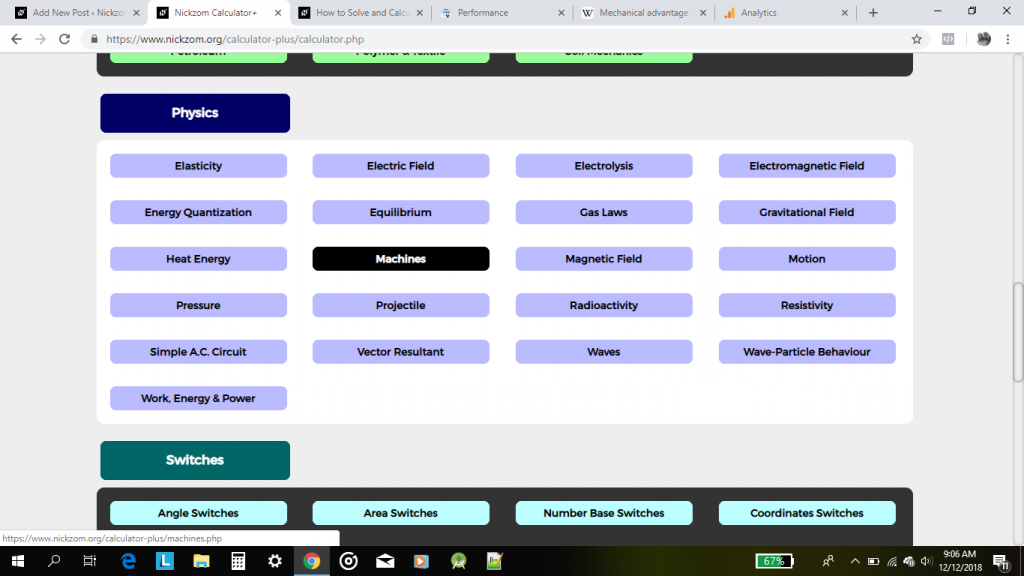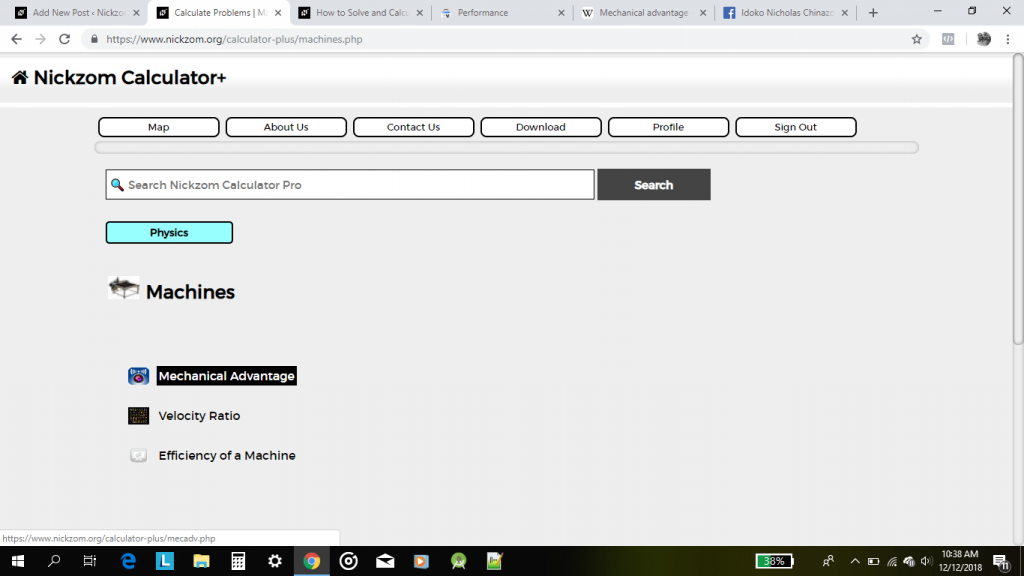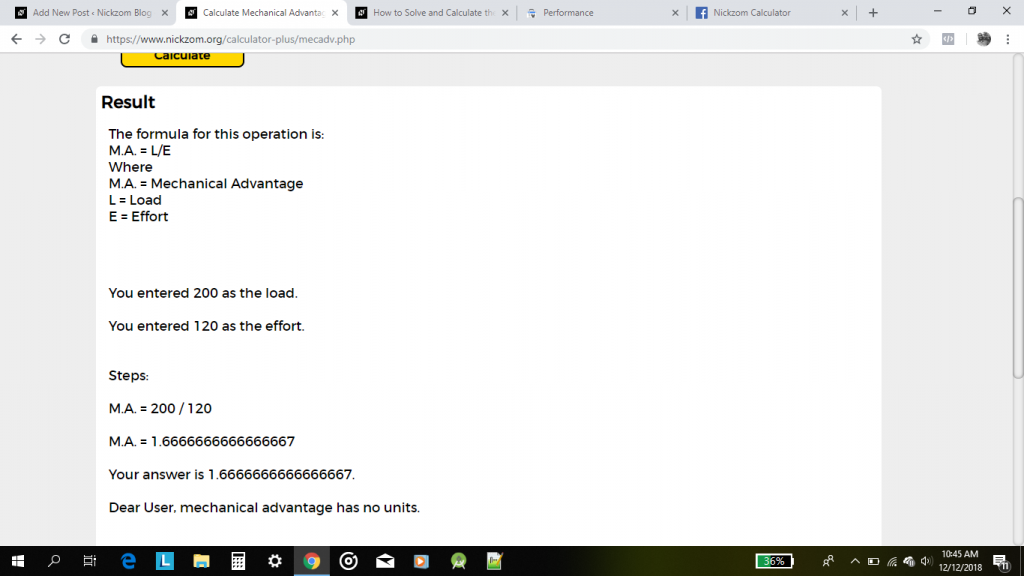# How to Calculate the Mechanical Advantage of a Machine (Physics)

Mechanical advantage is a very important parameter of machines and is considered a lever problem.

Mechanical advantage is the ratio of load to effort.

Nickzom Calculator – The Calculator Encyclopedia solves for mechanical advantage alongside a bunch of other problems in Physics.

For Example: Let’s calculate mechanical advantage of a machine when the load is 200 N and the effort is 120 N.

Mechanical Advantage = Load / Effort

Load = 200 N

Effort = 120 N

Mechanical Advantage = 200 / 120 = 1.6667

Therefore, the mechanical advantage of the machine is 1.6667.

Let me show you how Nickzom Calculator solves the mechanical advantage of a machine and displays the steps too.

First and Foremost, proceed to the Calculator Map. Then, click on Machines under Physics.Now, click on Mechanical Advantage in the Machines PageAn interface for you to enter the values of Load and Effort accordingly is displayed for you.Enter the appropriate valuesLastly, click on CalculateThe result of the calculation alongside the formula, parameter, steps and answer is displayed for you.

You can access Nickzom Calculator (The Calculator Encyclopedia) via any of these channels: